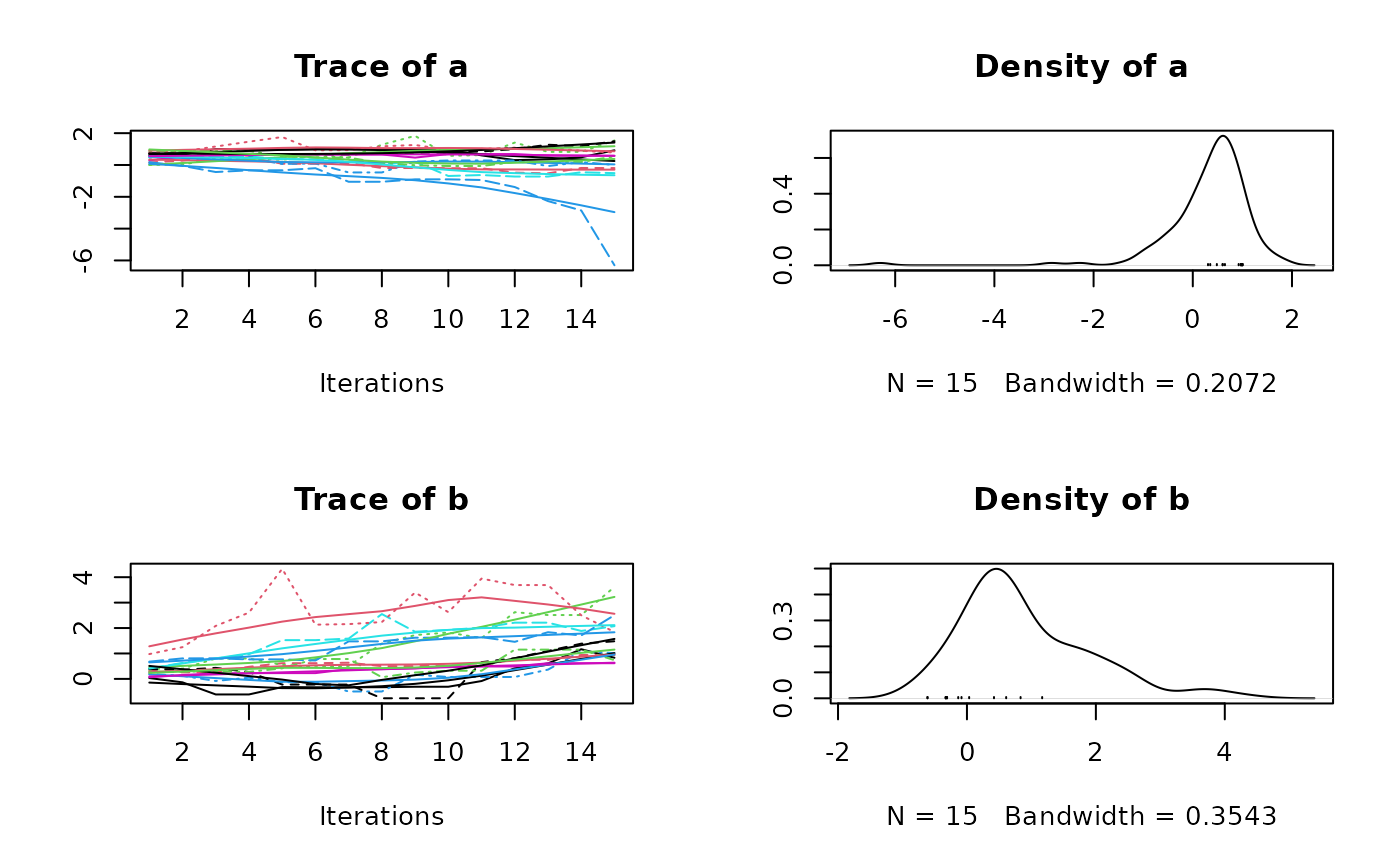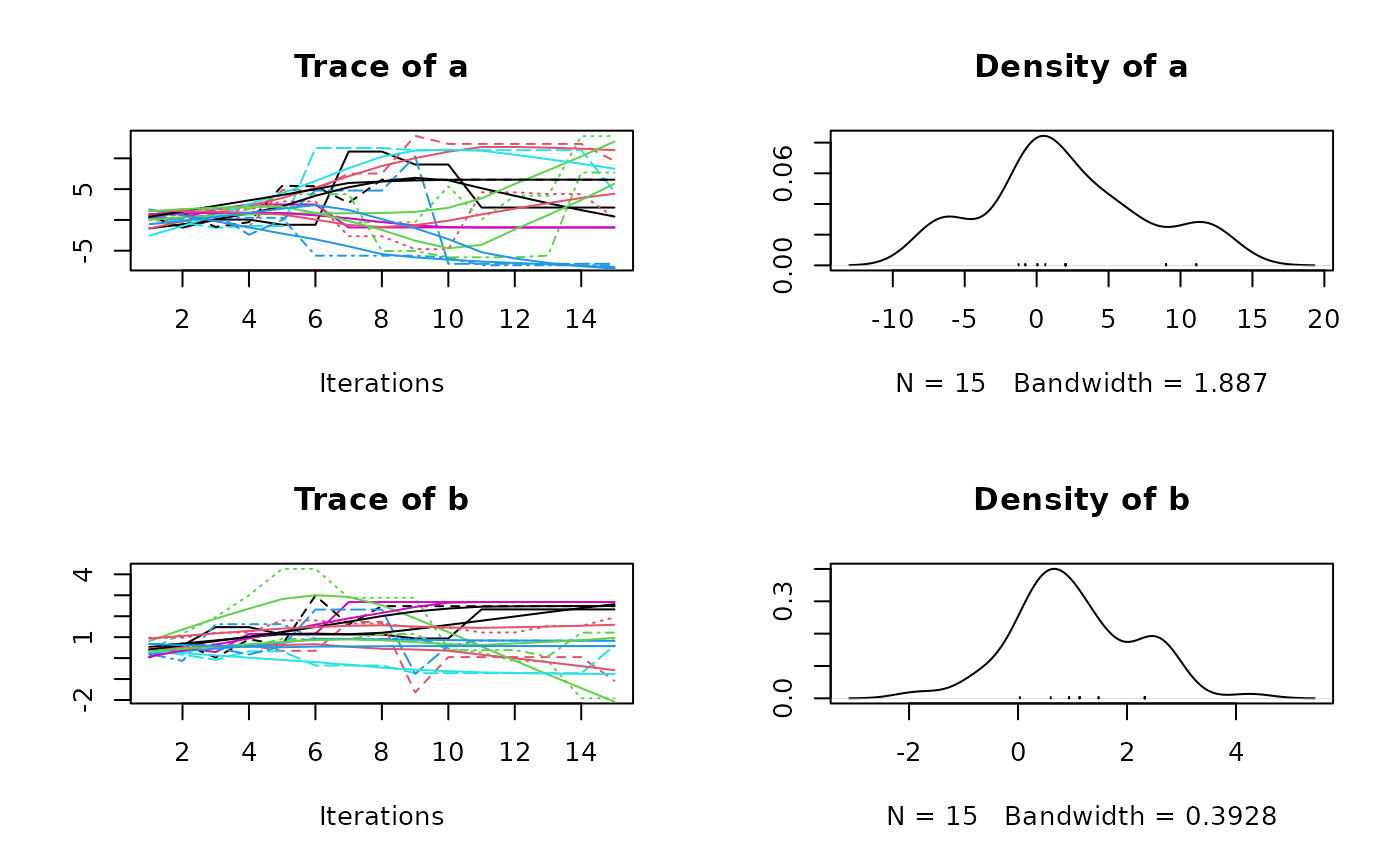Ensemble Markov Chain Monte Carlo sampler with different strategies to generate proposals. Either the stretch move as proposed by Goodman and Weare (2010), or a differential evolution jump move similar to Braak and Vrugt (2008).

MCMCEnsemble(
f,
inits,
max.iter,
n.walkers = 10 * ncol(inits),
method = c("stretch", "differential.evolution"),
coda = FALSE,
...
)

## Arguments

f

function that returns a single scalar value proportional to the log probability density to sample from.

inits

A matrix (or data.frame) containing the starting values for the walkers. Each column is a variable to estimate and each row is a walker

max.iter

maximum number of function evaluations

n.walkers

number of walkers (ensemble size)

method

method for proposal generation, either "stretch", or "differential.evolution". This argument will be saved as an attribute in the output (see examples).

coda

logical. Should the samples be returned as coda::mcmc.list object? (defaults to FALSE)

...

further arguments passed to f

## Value

• if coda = FALSE a list with:

• samples: A three dimensional array of samples with dimensions walker x generation x parameter

• log.p: A matrix with the log density evaluate for each walker at each generation.

• if coda = TRUE a list with:

• samples: A object of class coda::mcmc.list containing all samples.

• log.p: A matrix with the log density evaluate for each walker at each generation.

In both cases, there is an additional attribute (accessible via attr(res, "ensemble.sampler")) recording which ensemble sampling algorithm was used.

## Examples

## a log-pdf to sample from
p.log <- function(x) {
B <- 0.03                              # controls 'bananacity'
-x^2/200 - 1/2*(x+B*x^2-100*B)^2
}

## set options and starting point
n_walkers <- 10
unif_inits <- data.frame(
"a" = runif(n_walkers, 0, 1),
"b" = runif(n_walkers, 0, 1)
)

## use stretch move
res1 <- MCMCEnsemble(p.log, inits = unif_inits,
max.iter = 300, n.walkers = n_walkers,
method = "stretch")
#> Using stretch move with 10 walkers.

attr(res1, "ensemble.sampler")
#>  "stretch"

str(res1)
#> List of 2
#>  $samples: num [1:10, 1:30, 1:2] 0.882 0.511 0.639 0.168 0.182 ... #> ..- attr(*, "dimnames")=List of 3 #> .. ..$ : chr [1:10] "walker_1" "walker_2" "walker_3" "walker_4" ...
#>   .. ..$: chr [1:30] "generation_1" "generation_2" "generation_3" "generation_4" ... #> .. ..$ : chr [1:2] "a" "b"
#>  $log.p : num [1:10, 1:30] -2.94 -4.46 -3.94 -2.92 -3.67 ... #> ..- attr(*, "dimnames")=List of 2 #> .. ..$ : chr [1:10] "walker_1" "walker_2" "walker_3" "walker_4" ...
#>   .. ..$: chr [1:30] "generation_1" "generation_2" "generation_3" "generation_4" ... #> - attr(*, "ensemble.sampler")= chr "stretch" ## use stretch move, return samples as 'coda' object res2 <- MCMCEnsemble(p.log, inits = unif_inits, max.iter = 300, n.walkers = n_walkers, method = "stretch", coda = TRUE) #> Using stretch move with 10 walkers. attr(res2, "ensemble.sampler") #>  "stretch" summary(res2$samples)
#>
#> Iterations = 1:30
#> Thinning interval = 1
#> Number of chains = 10
#> Sample size per chain = 30
#>
#> 1. Empirical mean and standard deviation for each variable,
#>    plus standard error of the mean:
#>
#>      Mean    SD Naive SE Time-series SE
#> a -0.1991 4.823   0.2785         0.7742
#> b  0.8391 1.022   0.0590         0.1636
#>
#> 2. Quantiles for each variable:
#>
#>      2.5%     25%    50%   75%  97.5%
#> a -8.6060 -2.7948 0.1823 1.143 11.409
#> b -0.6609  0.1799 0.7476 1.366  3.529
#>
plot(res2$samples)## use different evolution move, return samples as 'coda' object res3 <- MCMCEnsemble(p.log, inits = unif_inits, max.iter = 300, n.walkers = n_walkers, method = "differential.evolution", coda = TRUE) #> Using differential.evolution move with 10 walkers. attr(res3, "ensemble.sampler") #>  "differential.evolution" summary(res3$samples)
#>
#> Iterations = 1:30
#> Thinning interval = 1
#> Number of chains = 10
#> Sample size per chain = 30
#>
#> 1. Empirical mean and standard deviation for each variable,
#>    plus standard error of the mean:
#>
#>    Mean    SD Naive SE Time-series SE
#> a 1.120 6.100  0.35220         1.0335
#> b 1.719 1.679  0.09695         0.1838
#>
#> 2. Quantiles for each variable:
#>
#>      2.5%     25%    50%   75% 97.5%
#> a -12.603 -2.5388 0.6322 4.458 13.02
#> b  -1.869  0.7428 2.0092 2.822  4.49
#>
plot(res3\$samples)